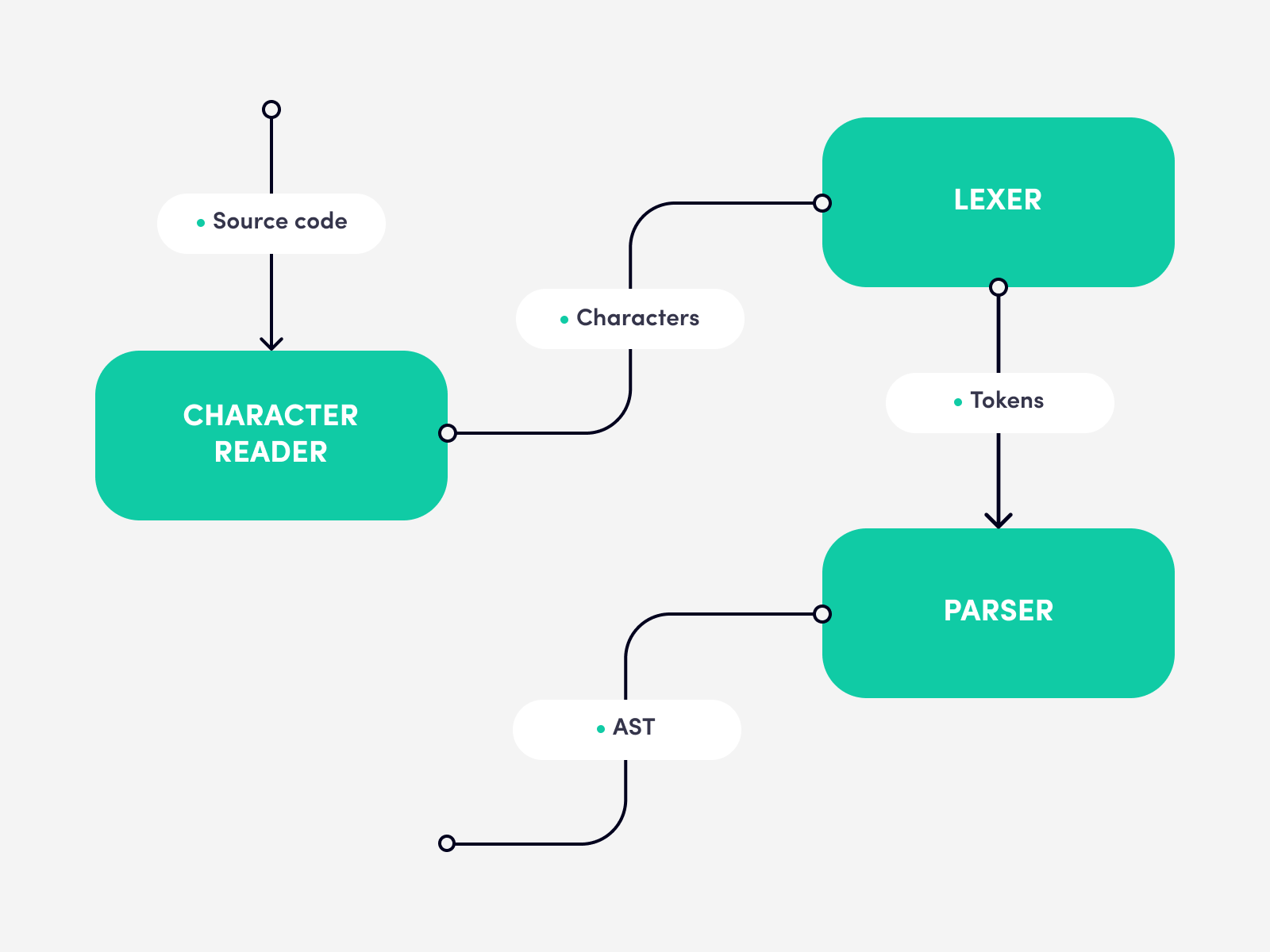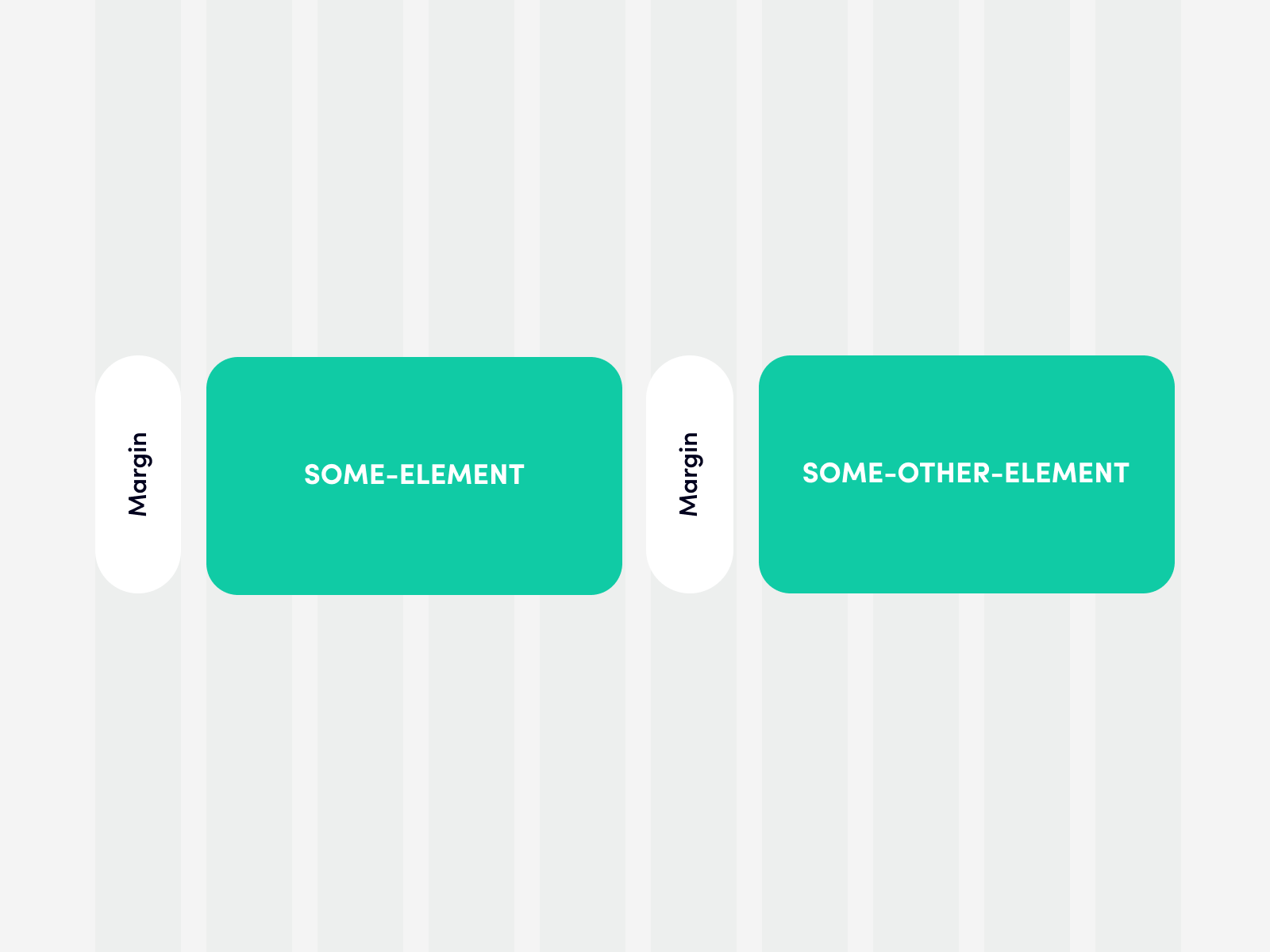## So, what is this?

SassScript is a set of extensions that can be included in the Sass documents to compute variables from property values and uses properties of variables, arithmetic, and other functions. That gives us the ability to organize a code into functions, perform conditional operations, and many more. Actually, these two abilities are enough to build a whole program.

## Let’s start

Typical parser implementations theory defines using a character reader, which is used by a lexer that produces tokens, and the last one is the parser itself which creates the AST.We will build a less capable compiler because Sass has some limitations. Sass cannot work with a filesystem in any way. Because of that, we cannot store custom syntax in separate files. So we should write it inside Sass files. Also, it is inconvenient to process a character's stream because Sass does not provide the most valuable methods for working with strings (and it does not have streams at all). The same goes for the lexer. But we can simulate the latter if we assume that all keywords are already tokens. Sass has a list structure where a space can be a separator between items. And there is a sass:list module that provides some functions to work with lists. We are going to use that ability to simulate the lexer skipping the character sequence stream at all. All the code we are going to write as an argument to a value function. And despite the length of the words, it will still be the list!

``value(sequence of the tokens);``

Let me describe the idea.

We plan to create a simple responsive grid system. For that, we will assume that there is a table with 64 columns (there may be more or less, it depends) which covers a whole page. Each cell of the table is a square figure. It gives us responsiveness because, on mobile phones, cells will have a smaller size than on laptops. Based on that, we receive a dynamic value (one side of the cell) that we use to express all dimensions in our grid.

You may want to use rem or vw for that purpose. And that’s okay. It’s up to you how to define a unit value. With that, we’ll be able to say, for example, that “this block should have a width of 5 columns“, and blocks will resize according to unit value changes.

Okay, let’s jump into the code now.

The simplest thing is to calculate the unit value. We know that the grid will have 64 columns. So, the width of one cell can be defined like this:

``````@use 'sass:math';

:root {
-—unit: math.div(100vw, 64);
}``````

Later, I won’t write imports of standard Sass’s modules for brevity. Assume that they are present already.

We took a benefit from CSS variables here and made it global. That will allow us to access this value everywhere in the runtime. We can place it into the mixin named init to allow the developer to override the columns count.

``````@mixin init(\$columns: 64) {
:root {
—-unit: math.div(100vw, #{\$columns});
}
}``````

Also, you can define lower and upper bounds for the unit value by using clamp CSS function, but we won’t do that to simplify the code examples.

Move on to the syntax. We're expecting our grid will work for the following use-cases:

``````value(8); // Just the columns count
value(from 6 to 21); // A distance from 6th column to the 21th column
value(8 min 20px max 70px); // The columns count with the lower and upper bounds of the result value
value(from 6 to 21 min 20px max 70px); // The same but with a range between columns``````

We have defined the two blocks of the expression: dimension and bounds. The latter is optional. Let’s dive deep into the dimension block a little bit.

The simplest case is when it equals the number - the columns count (see above). There is no more to discuss. The next variant is more difficult. It includes two mandatory keywords: from and to, and two interchangeable: start and end. The last two keywords equal to the 0 and 64 (or whatever number you decided to use) accordingly: from start to end. That expression is equal to from 0 to 64 or 100vw. The next block has only one variant: minmax. As numbers, you can use whatever value you want: pixels, rems, percentages, and so on.

That’s all. Now, when we have all rules defined, we shall go to the most exciting part - the code.

I already said that we would operate on the list of tokens, and for that purpose, we will use the list structure. Unfortunately, there are not enough methods to work with lists in SassScript. So, let’s implement missing ones. Firstly, we need the isEmpty function to determine whether a list has tokens or not.

``````@function isEmpty(\$list) {
@return list.length(\$list) == 0;
}``````

That function is pretty straightforward and does not require additional explanation.

Along with that, we should be able to remove processed tokens from the list. For that, we are going to implement a generic slice function.

``````@function slice(\$list, \$from, \$to: list.length(\$list) + 1) {
\$_separator: list.separator(\$list);
\$_copy: ();

@for \$index from \$from to \$to {
\$_copy: list.append(\$_copy, list.nth(\$list, \$index), \$_separator);
}

@return \$_copy;
}``````

Fun fact: all functions that are implemented in SassScript are pure.

It reminds the code from JavaScript with the difference that the first index of the list is 1, unlike 0 in JavaScript. Also, we should preserve a separator of an original list to the sliced, and that’s all.

With that, we can start writing our main value function.

``````@function value(\$tokens) {
\$_copy: \$tokens;
\$_numbers: (0, 0);
\$_borders: ();

@if isNumber(list.nth(\$_copy, 1)) {
\$_numbers: (0, number(\$_copy));
\$_copy: slice(\$_copy, 2);
} @else {
\$_numbers: range(\$_copy);
\$_copy: slice(\$_copy, 5);
}

@if not isEmpty(\$_copy) {
\$_borders: borders.borders(\$_copy);
}

@if not isEmpty(\$_borders) {
@return clamp(#{list.nth(\$_borders, 1)}, calc(var(--unit) * #{list.nth(\$_numbers, 2) - list.nth(\$_numbers, 1)}), #{list.nth(\$_borders, 2)});
}

@return calc(var(--unit) * #{list.nth(\$_numbers, 2) - list.nth(\$_numbers, 1)});
}``````

Let’s stop here. That is a huge function. Because the syntax is tiny and unambiguous, we can rely on the order of tokens. We know that first is the dimension block, and the second is the borders block. The first if/else block has the code for parsing tokens of the dimension block. Also, you saw \$_numbers and \$_borders variables. We could define them later, but it is better to define them with a default value to avoid possible errors while using it.

You may notice that there are unknown functions: isNumber, number and range. Let’s define them.

``````@function isNumber(\$value) {
@return meta.type-of(\$value) == "number";
}

@function number(\$list) {
\$_value: list.nth(\$list, 1);

@if not isNumber(\$_value) {
@error "#{\$_value} is not a number!";
}

@return \$_value;
}``````

isNumber, as it states from the name, checks if the value has a number type. number function takes a first token from the list and makes sure that it is a valid number.

The range function is a bit complex.

``````@function range(\$list) {
\$_copy: skip(from, \$list);
\$leftNumber: toNumber(list.nth(\$_copy, 1));
\$_copy: skip(to, slice(\$_copy, 2));
\$rightNumber: toNumber(list.nth(\$_copy, 1));

@if \$leftNumber > \$rightNumber {
@error 'From number cannot be greater than To';
}

@return (\$leftNumber \$rightNumber + 1);
}``````

Simply put, this function reads tokens and checks if they are valid. There are yet other functions that we should provide: skip and toNumber.

``````@function toNumber(\$value) {
@if \$value == keywords.\$start {
@return 0;
}

@if \$value == end {
@return unit.\$columns;
}

@if isNumber(\$value) {
@return \$value;
}

@error '#{\$value} is not a number or "#{keywords.\$start}"/"#{keywords.\$end}" keywords.';
}

@function skip(\$word, \$list) {
@if \$word != list.nth(\$list, 1) {
@error 'Word #{\$word} does not match the #{\$list} sequence.';
}

@return slice(\$list, 2);
}``````

toNumber function is needed to convert start and end keywords to corresponding numbers and check whether the starting column’s value is lower than the ending column’s value. skip is just a convenient method to skip the first token in the list.

range should return starting and ending column numbers to the value to calculate an actual value. Okay, the dimension block is ready. The borders block remains only.

``````@function borders(\$tokens) {
\$_copy: \$tokens;
\$_min: 0;
\$_max: \$_min;

\$_copy: skip(min, \$_copy);

\$_min: list.nth(\$_copy, 1);

\$_copy: slice(\$_copy, 2);

\$_copy: skip(max, \$_copy);

\$_max: list.nth(\$_copy, 1);

@return (\$_min, \$_max);
}``````

Here the logic is the same as in the range function. At that point, we have a complete tiny compiler though it looks somewhat different than typical implementations. But the primary goal of this article is to show that Sass is much more powerful than it seems, and even though CSS gets many features that Sass has, the latter is still in demand.

Now, we can use our super compiler to write dimensions in the 64 columns' system. The example of the code:

``````@use 'grid' as *;

.some-element {
margin-left: value(1);
width: value(from 1 to 8);
height: value(3);
background-color: tomato;
}

.some-other-element {
width: value(from 10 to 16);
margin-left: value(1);
height: value(3);
background-color: tomato;
}``````

That gives us simpler management of an element’s position. Thus it can be used as a simple replacement for the common grid systems. Also, it can help you to be closer to design because designers use similar grids to combine elements into the whole page.

Personally, it reminds me of a mix of Bootstrap and Susy:The further development of that idea you can see here. There is an example page that shows simple elements positioning inside the grid. We implemented that idea on our site, that's why we can say it works and works well.

Thank you for reading, and have fun!